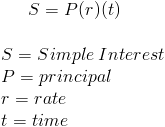# Simple InterestThe simple interest formula is used to calculate the interest accrued on a loan or savings account that has simple interest. The simple interest formula is fairly simple to compute and to remember as principal times rate times time. An example of a simple interest calculation would be a 3 year saving account at a 10% rate with an original balance of \$1000. By inputting these variables into the formula, \$1000 times 10% times 3 years would be \$300.

Simple interest is money earned or paid that does not have compounding. Compounding is the effect of earning interest on the interest that was previously earned. As shown in the previous example, no amount was earned on the interest that was earned in prior years.

As with any financial formula, it is important that rate and time are appropriately measured in relation to one another. If the time is in months, then the rate would need to be the monthly rate and not the annual rate.

## Ending Balance with Simple Interest Formula

The ending balance, or future value, of an account with simple interest can be calculated using the following formula:Using the prior example of a \$1000 account with a 10% rate, after 3 years the balance would be \$1300. This can be determined by multiplying the \$1000 original balance times [1+(10%)(3)], or times 1.30.

Instead of using this alternative formula, the amount earned could be simply added to the original balance to find the ending balance. Still using the prior example, the calculation of the formula that is on the top of the page showed \$300 of interest. By adding \$300 to the original amount of \$1000, the result would be \$1300.

New to Finance?